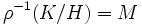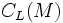# Direct factor implies right-quotient-transitively central factor

This article gives the statement and possibly, proof, of an implication relation between two subgroup properties. That is, it states that every subgroup satisfying the first subgroup property (i.e., direct factor) must also satisfy the second subgroup property (i.e., right-quotient-transitively central factor)
View all subgroup property implications | View all subgroup property non-implications
Get more facts about direct factor|Get more facts about right-quotient-transitively central factor

## Statement

### Statement with symbols

Suppose$G$ is a group,$H \le K \le G$, and$H$ is a direct factor of$G$. Suppose, further, that$K/H$ is a central factor of$G/H$. Then,$K$ is a central factor of$G$.

## Proof

Given: A group$G$, a direct factor$H$ of$G$,$K$ contains$H$ and$K/H$ is a central factor of$G/H$.

To prove:$K$ is a central factor of$G$.

Proof: Since$H$ is a direct factor of$G$, there exists a normal complement$L$ to$H$ in$G$, with$HL = G$ and$H \cap L$ trivial. Let$M = K \cap L$.

Consider the map$\rho:L \to G/H$ that sends every element of$L$ to its$H$-coset in$G$. This map is an isomorphism, since the kernel$H \cap L$ is trivial, and$\rho^{-1}(K/H) = M$. Thus,$HM = K$.

1. Every element of$H$ centralizes every element of$L$: Since both$H$ and$L$ are normal in$G$,$[H,L]$ is contained in both, and since they intersect trivially,$[H,L]$ is trivial, so every element of$H$ centralizes every element of$L$.
2.$MC_L(M) = L$: Since$\rho$ is an isomorphism, and$\rho(M)$ is a central factor of$G/H$,$M$ is a central factor of$L$.
3.$C_L(M)$ centralizes$K$, i.e.,$C_L(M) \le C_G(K)$: By step (1),$C_L(M)$ centralizes$H$, since$L$ centralizes$H$. It also centralizes$M$ by definition. Thus, it centralizes$HM = K$.
4.$KC_L(M) = G$: We have$KC_L(M) = HMC_L(M) = HL = G$.
5.$KC_G(K) = G$: This follows from the previous two steps.

This completes the proof.